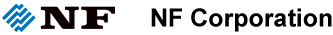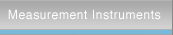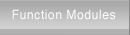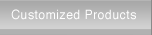home > Products > Measurement Instruments > Impedance Analyzer > ZGA5920 > Measurement & AnalysisSignal GeneratorLCR MeterImpedance AnalyzerAC Voltmeter/Noise MeterLock-in Amplifier/PreamplifierFilterMeasurement SystemFrequency Response AnalyzerProduct InformationFeaturesMeasurement & AnalysisSpecificationsPeripheral EquipmentImpedance/Gain-Phase Analyzer ZGA5920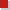Measurement and AnalysisList of measurement and analysis parameters (690 KB)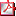Electronic materialsMeasurement parameters: Admittance, phase, conductance, susceptanceAnalysis and simulation: Parameter extraction, matching inductance, parameter simulationMeasurement parameters: Parallel capacitance, parallel resistanceAnalysis and simulation: Dielectric permittivity, dissipation factorMeasurement parameters: Series self-inductance, Series resistanceAnalysis and simulation: Magnetic permittivity, Dissipation factorElectronic and electrical componentsMeasurement parameters: Series self-inductance, parallel self-inductance, series resistance, parallel resistance, phase, quality factorAnalysis and simulation: Equivalent circuit estimation and simulationMeasurement parameters: Series capacitance, parallel capacitance, series resistance, phase, dissipation factor, quality factorAnalysis and simulation: Equivalent circuit estimation and simulationMeasurement parameters: Impedance, resistance, reactance, phaseAnalysis and simulation: Equivalent circuit estimation and simulationMeasurement parameters: Leakage inductance, turn ratioAnalysis and simulation: Mutual inductance, coupling coefficient,Measurement parameters: CV characteristics, parallel capacitance, quality factorAnalysis and simulation: Tuning characteristics simulationElectronic circuitsMeasurement parameters: Loop gain characteristics, closed loop characteristics, open loop characteristics, feedback transfer functionAnalysis and simulation: Phase margin, gain margin, circuit model identification, circuit model simulationMeasurement parameters: Gain-phase characteristics, CMRR characteristics, PSRR characteristics, differential gain/phase characteristic, saturation characteristicsAnalysis and simulation: Transfer function identification, transfer function simulationMeasurement parameters: Frequency characteristicsAnalysis and simulation: Transfer function identification, transfer function simulation